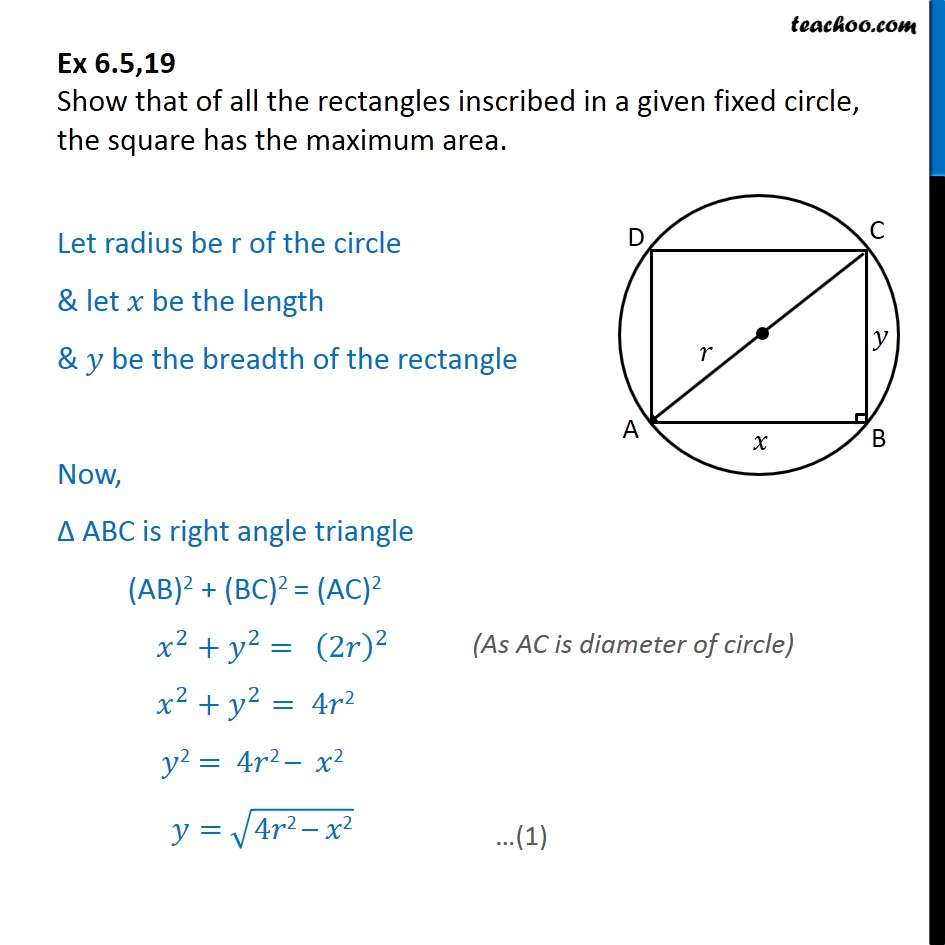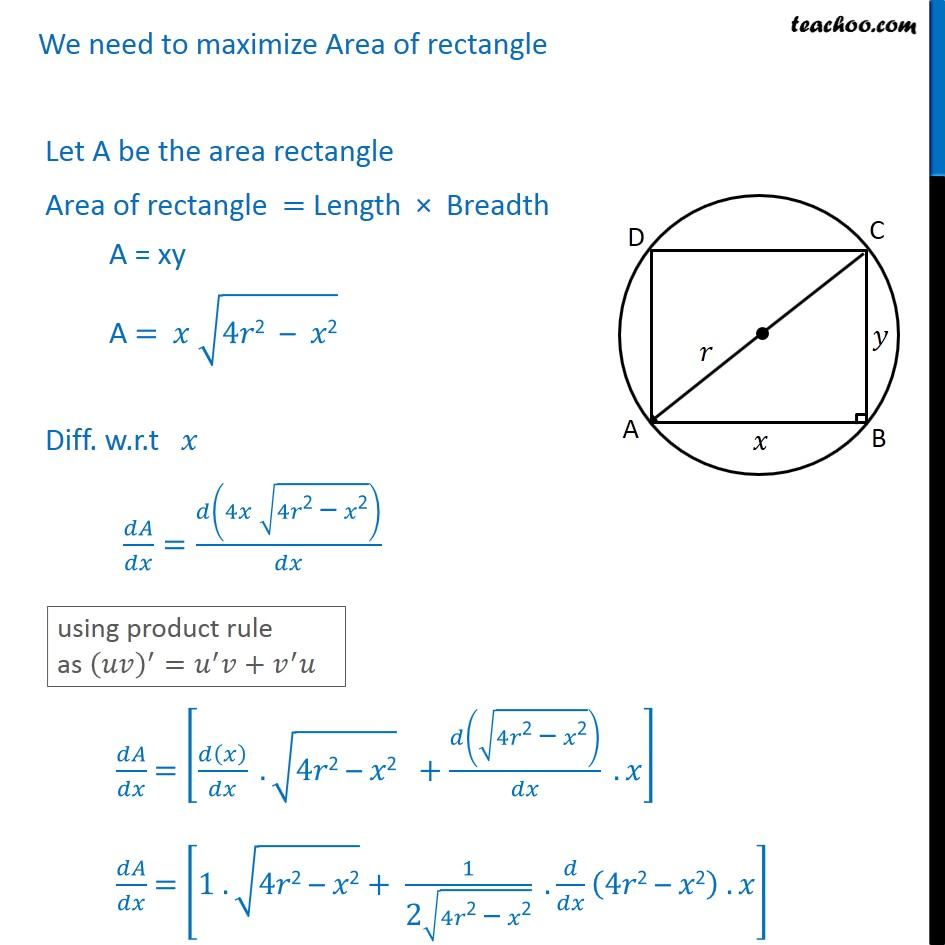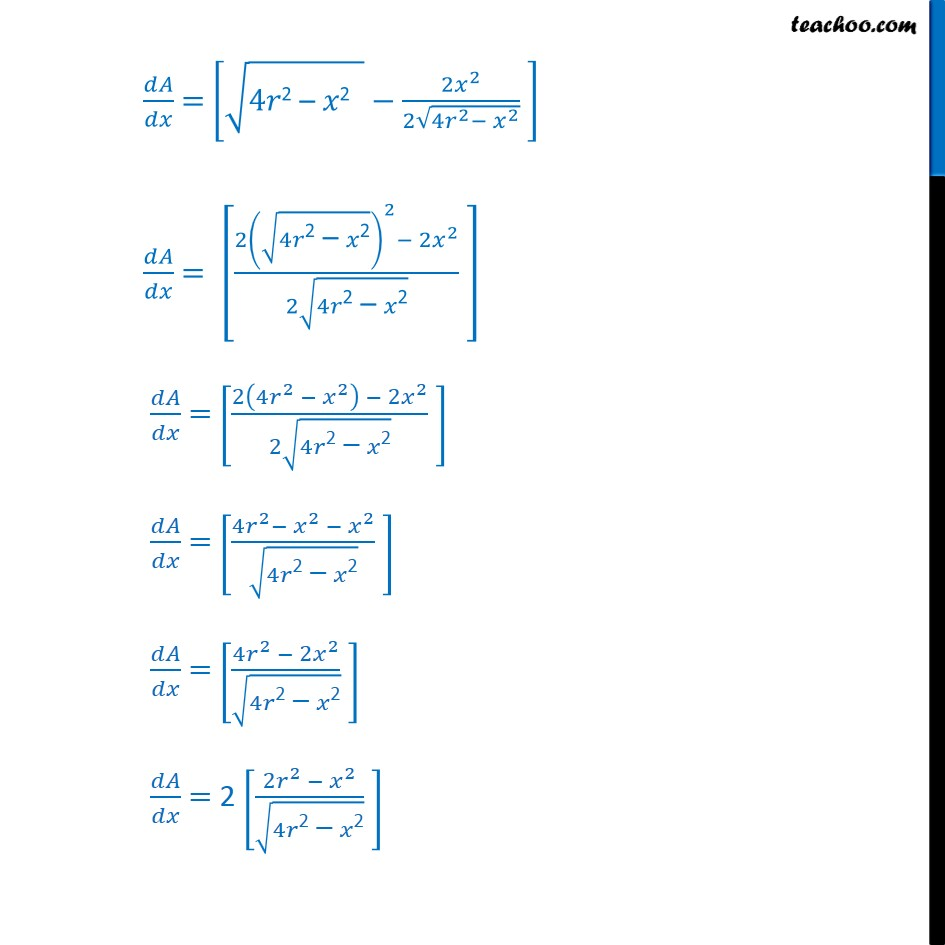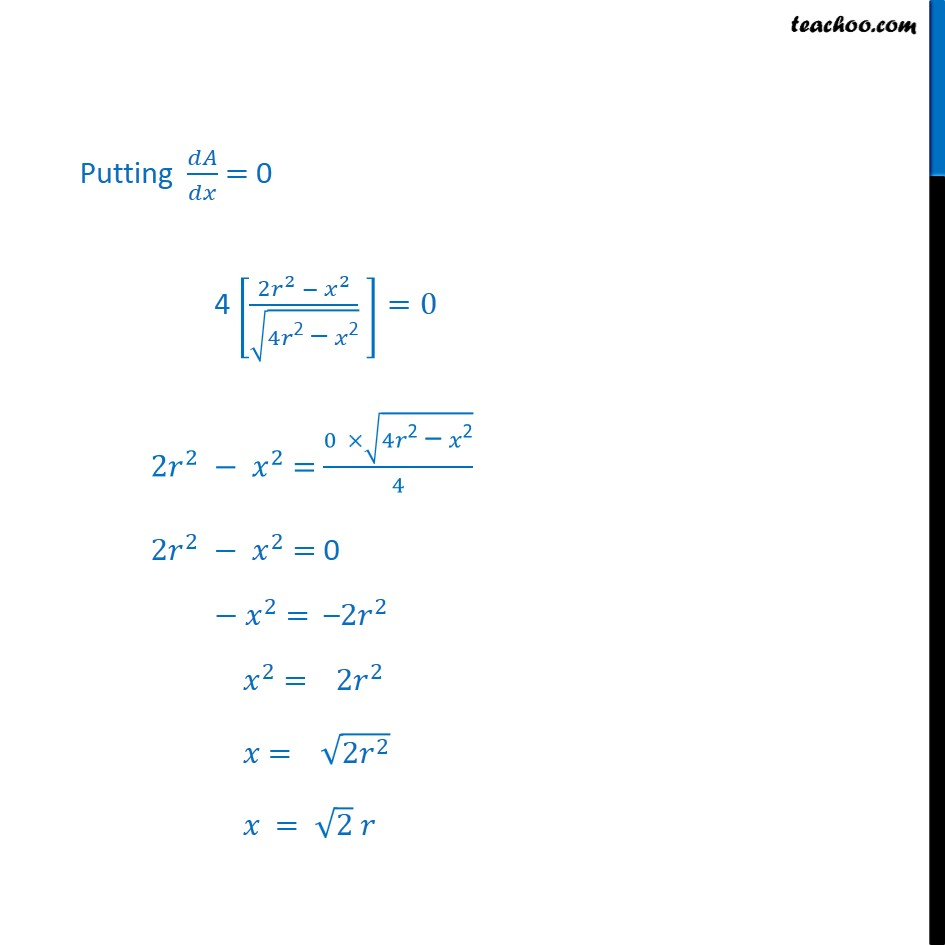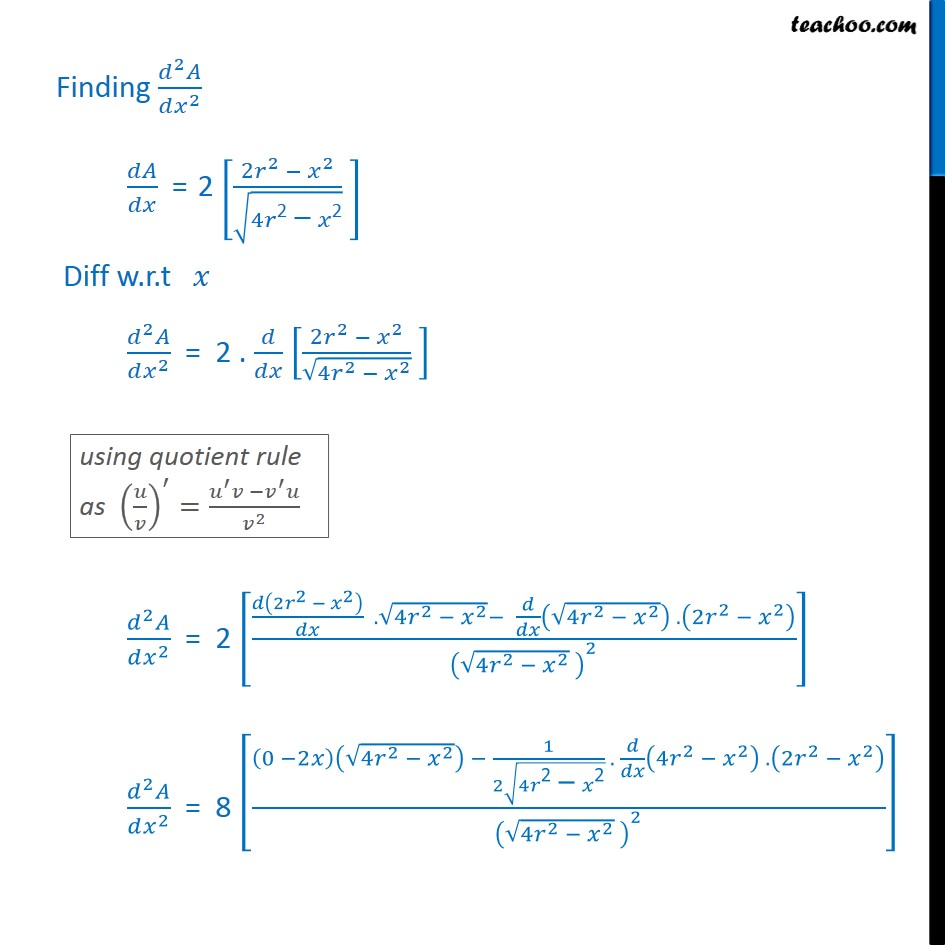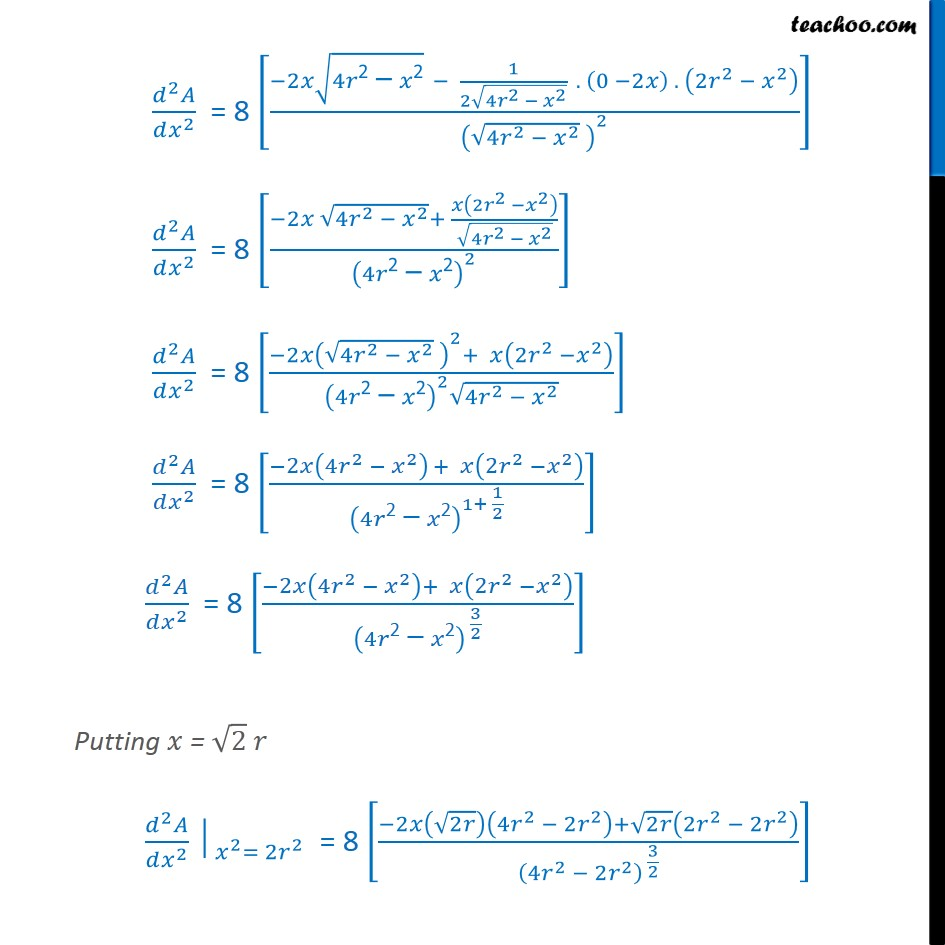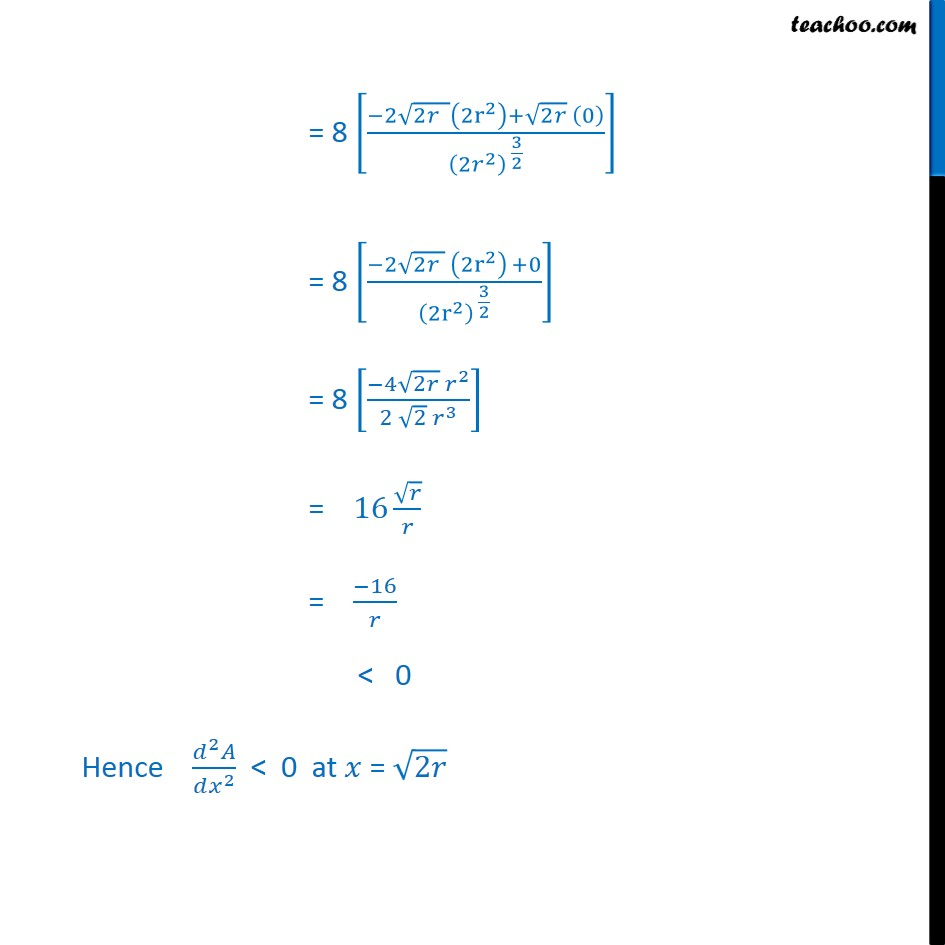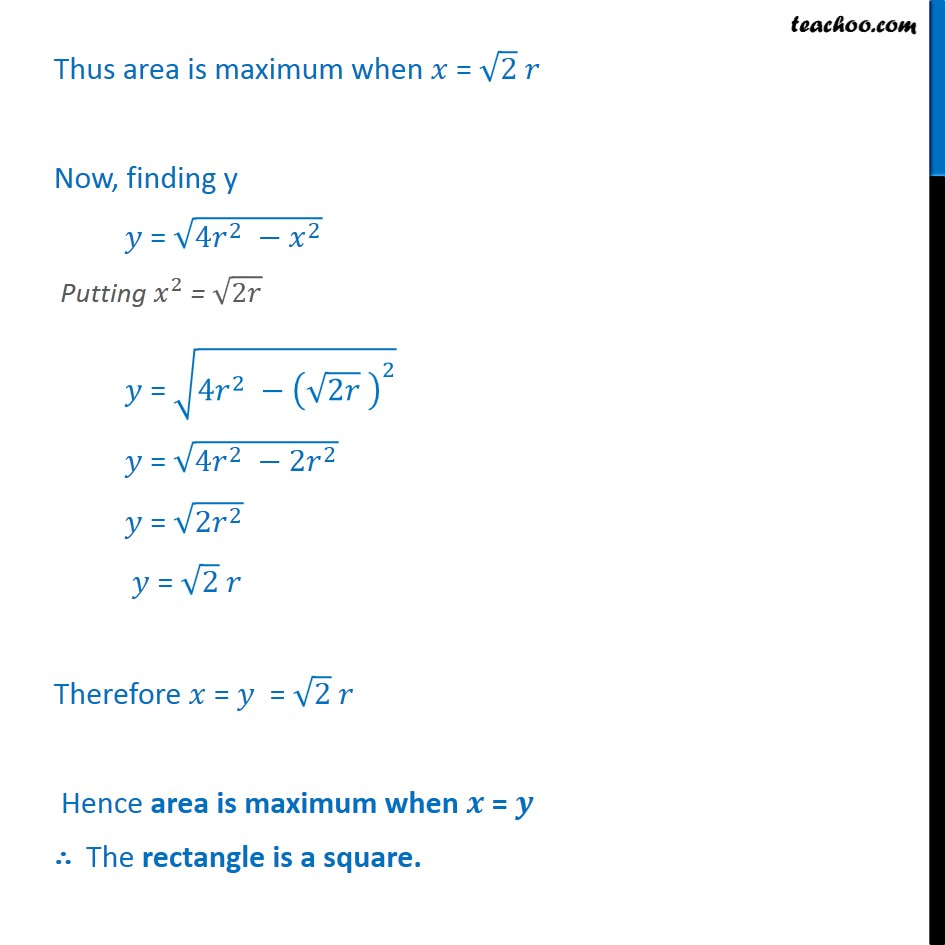1. Chapter 6 Class 12 Application of Derivatives
2. Serial order wise
3. Ex 6.5

Transcript

Ex 6.5,19 Show that of all the rectangles inscribed in a given fixed circle, the square has the maximum area. Let radius be r of the circle & let be the length & be the breadth of the rectangle Now, ABC is right angle triangle (AB)2 + (BC)2 = (AC)2 2 + 2 = 2 2 2 + 2 = 4 2 2 = 4 2 2 = 4 2 2 We need to maximize Area of rectangle Let A be the area rectangle Area of rectangle = Length Breadth A = xy A = 4 2 2 Diff. w.r.t = 4 4 2 2 = . 4 2 2 + 4 2 2 . = 1 . 4 2 2 + 1 2 4 2 2 . 4 2 2 . = 4 2 2 2 2 2 4 2 2 = 2 4 2 2 2 2 2 2 4 2 2 = 2 4 2 2 2 2 2 4 2 2 = 4 2 2 2 4 2 2 = 4 2 2 2 4 2 2 = 2 2 2 2 4 2 2 Putting = 0 4 2 2 2 4 2 2 =0 2 2 2 = 0 4 2 2 4 2 2 2 = 0 2 = 2 2 2 = 2 2 = 2 2 = 2 Finding 2 2 = 2 2 2 2 4 2 2 Diff w.r.t 2 2 = 2 . 2 2 2 4 2 2 2 2 = 2 2 2 2 . 4 2 2 4 2 2 . 2 2 2 4 2 2 2 2 2 = 8 0 2 4 2 2 1 2 4 2 2 . 4 2 2 . 2 2 2 4 2 2 2 2 2 = 8 2 4 2 2 1 2 4 2 2 . 0 2 . 2 2 2 4 2 2 2 2 2 = 8 2 4 2 2 + 2 2 2 4 2 2 4 2 2 2 2 2 = 8 2 4 2 2 2 + 2 2 2 4 2 2 2 4 2 2 2 2 = 8 2 4 2 2 + 2 2 2 4 2 2 1+ 1 2 2 2 = 8 2 4 2 2 + 2 2 2 4 2 2 3 2 Putting = 2 2 2 2 = 2 2 = 8 2 2 4 2 2 2 + 2 2 2 2 2 4 2 2 2 3 2 = 8 2 2 2 r 2 + 2 0 2 2 3 2 = 8 2 2 2 r 2 +0 2 r 2 3 2 = 8 4 2 2 2 2 3 = 16 = 16 < 0 Hence 2 2 < 0 at = 2 Thus area is maximum when = 2 Now, finding y = 4 2 2 Putting 2 = 2 = 4 2 2 2 = 4 2 2 2 = 2 2 = 2 Therefore = = 2 Hence area is maximum when = The rectangle is a square.

Ex 6.5